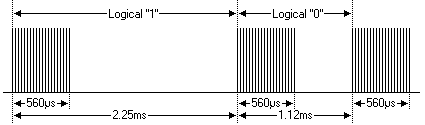Position: 艾鍗學院 Blog > 艾鍗文件區 > Embedded LInux 專欄Linux IR 解碼
by 艾鍗學院, 2014-02-10 23:30, Views(1572)NEC Infrared Protocol

• A 9ms leading pulse burst (16 times the pulse burst length used for a logical data bit)
• A 4.5ms space
• The 8-bit address for the receiving device
• The 8-bit logical inverse of the address
• The 8-bit command
• The 8-bit logical inverse of the command
• Final 562.5µs pulse burst to show end of message transmission.
• Logical '0' – a 562.5µs pulse burst followed by a 562.5µs space, with a total transmit time of 1.125ms
• Logical '1' – a 562.5µs pulse burst followed by a 1.6875ms space, with a total transmit time of 2.25

Pulse 係由很多 on/off 交替的訊號所組成:

• Carrier frequency = 38kHz
• duty factor = 1/3
• period = 26.5us16 bits for the address (address + inverse) require 27ms to transmit time .and the 16 bits for the command (command + inverse) also require 27ms to transmit time.
because (address + address inverse) or (command+command inverse) will always contain 8 '0's  and 8 '1's    so (8 * 1.125ms) + (8 * 2.25ms) == 27 ms .
according to this total time required to transmit the frame is (9ms +4.5ms +27ms+27ms) = 67.5 ms.

If the key on the remote controller is kept depressed, a repeat code will be issued, typically around 40ms after the pulse burst that signified the end of the message. A repeat code will continue to be sent out at 108ms intervals, until the key is finally released. The repeat code consists of the following, in order:

A 9ms leading pulse burst
A 2.25ms space
A 562.5µs pulse burst to mark the end of the space (and hence end of the transmitted repeat code).
the figures give blow show the timing of repeat codesif user keeps the key depressed the repeat codes keep coming

==========
```static irqreturn_t cir_irq_handler (int irq, void *dev_id, struct pt_regs *regs)
{
struct timeval tv;
long deltv;
int data;
struct cir_device_data *dev;

dev = (struct cir_device_data *)dev_id;

/* get current time */
do_gettimeofday(&tv);

if(dev->ir_state == IR_STATE_0) {
dev->ir_state = IR_STATE_1;
/* Initializate ir variable */
dev->ir_count = 0;
dev->complete_bits = 0;

/* Configure to generate an interrupt on rising edge
* Wait 9ms pre-pulse.
*/
set_irq_type(gpio_to_irq(CIR_GPIO), IRQT_RISING);
} else {
deltv = tv.tv_sec - dev->lasttv.tv_sec;

/* calc time since last interrupt in microseconds */
data = (int) (deltv*1000000 + tv.tv_usec - dev->lasttv.tv_usec);
decode_process(dev, data);
}

/* restore time */
dev->lasttv = tv;

return IRQ_HANDLED;
}```

```static int check_bit(int interval)
{
int bit = 0;

/* if interval is 1.12ms(+-20%) then bit 0 */
if(interval > 896 && interval < 1344)
bit = 0;
/* if interval is 2.25ms(+-20%) then bit 1 */
else if(interval > 1800 && interval < 2700)
bit = 1;
else
/* invalid data bit */
bit = -1;

return bit;
}

static void decode_process(struct cir_device_data *dev, int interval)
{
int bitvalue = 0;

switch(dev->ir_state){
case IR_STATE_1:
/* Configure to generate an interrupt on falling edge
* decision 9ms pre-pulse, within a +-20% range of the nominal value
* 9000 - (9000*0.2) = 7200, 9000+(9000*0.2) = 9900
*/
set_irq_type(gpio_to_irq(CIR_GPIO), IRQT_FALLING);
if(interval > 7200 && interval < 10800)
dev->ir_state = IR_STATE_2; // pre-pulse detected.
else
dev->ir_state = IR_STATE_0; // exit with error, return state_0
break;
case IR_STATE_2:
/* decision long interval(4.5ms) or short interval (2.25ms)
* long interval is normal message, short interval is repetition code.
* 4500 - (4500*0.2) = 3600, 4500 + (4500*0.2) = 5400
* 2250 - (2250*0.2) = 1800, 2250 + (2250*0.2) = 2700
*/
if(interval > 3600 && interval < 5400) {
dev->ir_state = IR_STATE_3;
} else if(interval > 1800 && interval < 2700) {
/* driver not supported repetition.
* Return state_0
*/
dev->ir_state = IR_STATE_0; //repeat state.
} else {
dev->ir_state = IR_STATE_0;     // exit with error, return state_0
printk(KERN_DEBUG "CIR:exit with error!\n");
}
break;
case IR_STATE_3:
/* 32 bits entire message receiving
*/
bitvalue = check_bit(interval);
if(bitvalue < 0) {
/* invalid data bit */
dev->ir_state = IR_STATE_0;
} else {
dev->complete_bits <<= 1;
dev->complete_bits |= bitvalue;
dev->ir_state = IR_STATE_3;
dev->ir_count++;

if(dev->ir_count >= CIR_DATA_BITS) {
/* receiving complete */
dev->ir_state = IR_STATE_0;
/* store to buffer */
dev->buff[dev->head] = dev->complete_bits;
dev->head = (dev->head + 1) % CIR_BUFFER_SIZE;
wake_up_interruptible(&dev->waitq);
}
}
break;
default:
break;
}
}```